### MEMO 2017 ekipno problem 5

Kvaliteta:
Avg: 0,0
Težina:
Avg: 6,0

Let$ABC$ be an acute-angled triangle with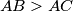$AB > AC$ and circumcircle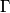$\Gamma$. Let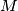$M$ be the midpoint of the shorter arc$BC$ of$\Gamma$, and let$D$ be the intersection of the rays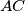$AC$ and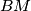$BM$. Let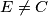$E \neq C$ be the intersection of the internal bisector of the angle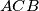$ACB$ and the circumcircle of the triangle$BDC$. Let us assume that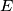$E$ is inside the triangle$ABC$ and there is an intersection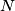$N$ of the line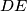$DE$ and the circle$\Gamma$ such that$E$ is the midpoint of the segment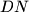$DN$.
Show that$N$ is the midpoint of the segment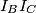$I_B I_C$, where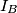$I_B$ and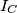$I_C$ are the excentres of$ABC$ opposite to$B$ and$C$, respectively.

Izvor: Srednjoeuropska matematička olimpijada 2017, ekipno natjecanje, problem 5